> 文档中心 > 【C++ Primer 5th】 第二章.变量与基本类型笔记 （数据类型 | 变量|指针|&|const|auto|typedef|decltype|头文件|预处理））

# 【C++ Primer 5th】 第二章.变量与基本类型笔记 （数据类型 | 变量|指针|&|const|auto|typedef|decltype|头文件|预处理））### 目录 or 思维导图

• 一、基本内置类型
• 复合类型
• ⽆符号类型
• 类型转化
• 含⽆符号类型的表达式
• 字面值常量
• 二、变量
• 概念
• 初始化
• 变量的定义与声明
• 标识符
• 作用域
• 三、复合类型
• 引⽤（reference）
• 指针（pointer）
• 指针值
• 空指针
• void *指针
• 指向指针的指针
• 指向指针的引用
• 四、const 限定符
• 初始化
• const的引⽤：常量引用
• 指针和const
• 顶层const & 底层const
• constexpr和常量表达式
• 五、处理类型
• typedef
• auto
• decltype
• 六、自定义数据结构
• 类型
• 类的使用
• 头文件 "xx.h"
• ==**?感谢阅读?**==

• 内容 ：
`数据类型`是程序的基础：它告诉我们数据的意义以及我们能在数据上执行的操作,并且数据类型决定了程序中数据和操作的意义。
`数据类型存在的意义`：给变量分配合适的内存空间

# 一、基本内置类型

C++定义了一套包括算术类型（arithmetic type）和空类型（void）在内的基本数据类型。其中算术类型包含了字符、整型数、布尔值和浮点数。空类型不对应具体的值。

## 复合类型

bit（比特）

Byte（字节）

`C++：算术类型`

bool 布尔类型 未定义
char 字符 8 位
wchar_t 宽字符 16 位
charl6_t Unicode 字符 16 位
char32_t Unicode 字符 32 位
short 短整型 16 位
int 整型 16 位
long 长整型 32 位
long long (C11) 长整型 64 位
float 单精度浮点数 7 位有效数字 （4字节）
double 双精度浮点数 15~16 位有效数字（8字节）
long double 扩展精度浮点数 10 位有效数字

`编译器浮点数类型默认显示小数点后6位 （这个数是小于1的情况下）`

## ⽆符号类型

`int、short、long和long long`都是带符号的，前面加上`unsigned`就可以得到无符 号类型，例如`unsigned long`
`unsigned int`可以缩写成`unsigned`
`char`比较特殊，类型分为三种：`char、signed char、unsigned char`
`char``signed char``unsigned char`的其中一种（编译器决定）
`8 byte``char` 范围：-128~127 ，`unsigned char `范围：0 ~ 255

①当明确知晓数值不可能为负时，选用无符号类型。
②使用int执行整数运算。在实际应用中，short常常显得太小而long一般和int有一样的尺寸。如果你的数值超过了int的表示范围，选用long long。
③在算术表达式(加减乘除)中不要使用char或bool，只有在存放字符或布尔值时才使用它们。因为char在不同编译器是不同的
④ 执行浮点数运算选用double，这是因为float通常精度不够而且\双精度运算甚至比单精度还快。long double提供的精度在一般情况下是没有必要的，况且它带来的运行时消耗也不容忽视。

## 类型转化

``bool b=42; //b为真(1)  bool的值非0即1  不宜这算术表达式用布尔值int i=1; //i == 1 int i=3.14;// i == 3 精度丢失double pi=i;//假设char占8比特，c的值为255unsigned char c=-1;//假设char占8比特，c的值为255signed char c2=256;//假设char占8比特，c2的值未定义,可能是垃圾值，也可能崩溃``

## 含⽆符号类型的表达式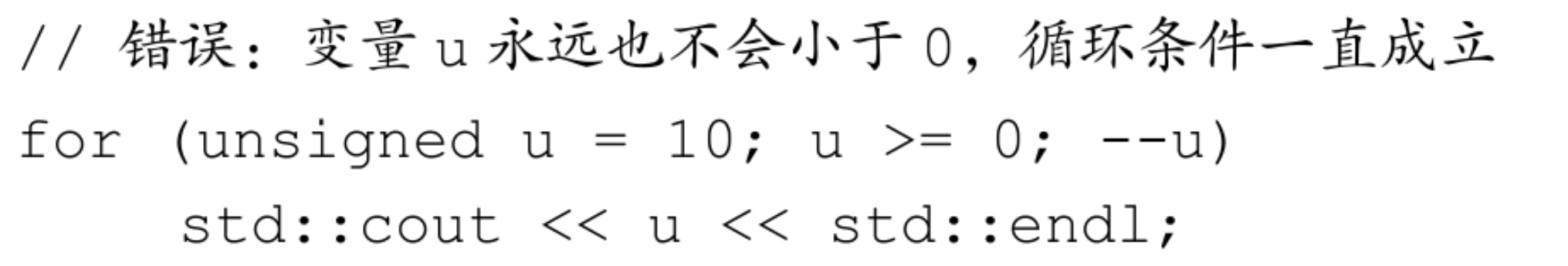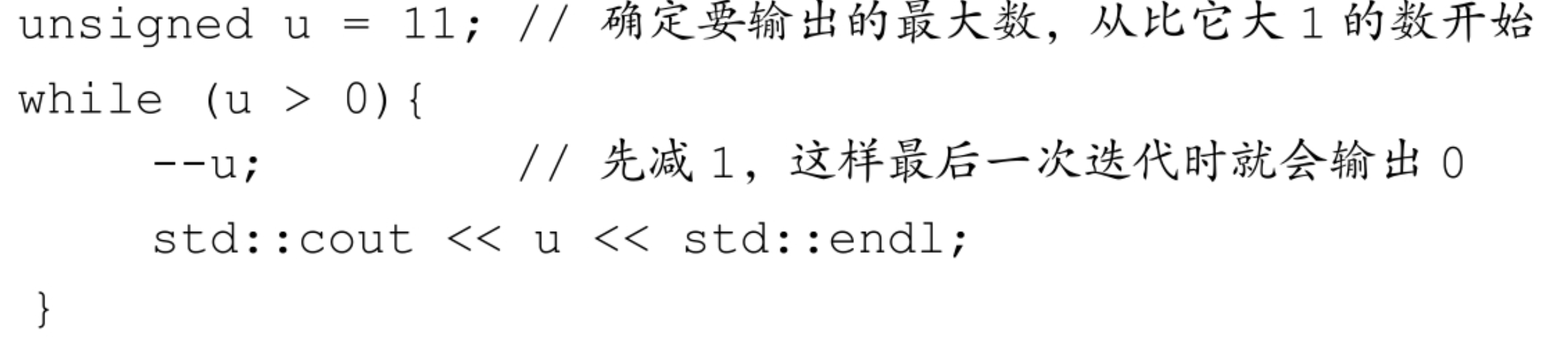``unsigned u=10;int i=-42; std::cout << i+i << std::endl;//输出-84 std::cout << u+i << std::endl;//如果int占32位，输出4294967264//2的32次方为4294967296 全是1表示的是-1，因为-1+1=0``

## 字面值常量

• 可以将整形写成十进制、八进制或十六进制• 浮点型字面值是一个double类型的值，表现为一个小数或科学计数法的指数形式 ：• 字符和字符串字面值

``'a'//字符字面值 "Hello World"//字符串字面值 /0结尾``

• 转义序列，C++定义的转义序列包括：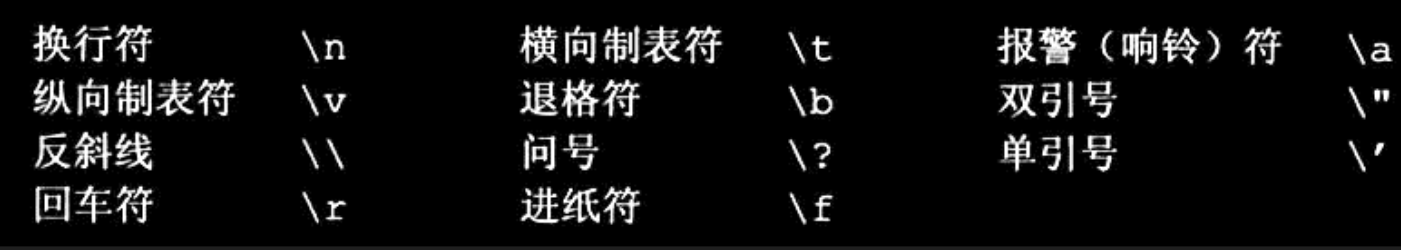• 泛化的转义序列，其形式是\x后紧跟1个或多个十六进制的数字，或、后面紧跟1/2 或3个八进制的数字。假设使用的是Latin-1字符集，以下是一些示例：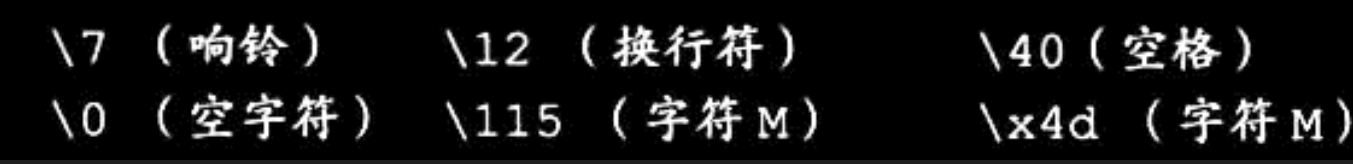• 指定字面值的类型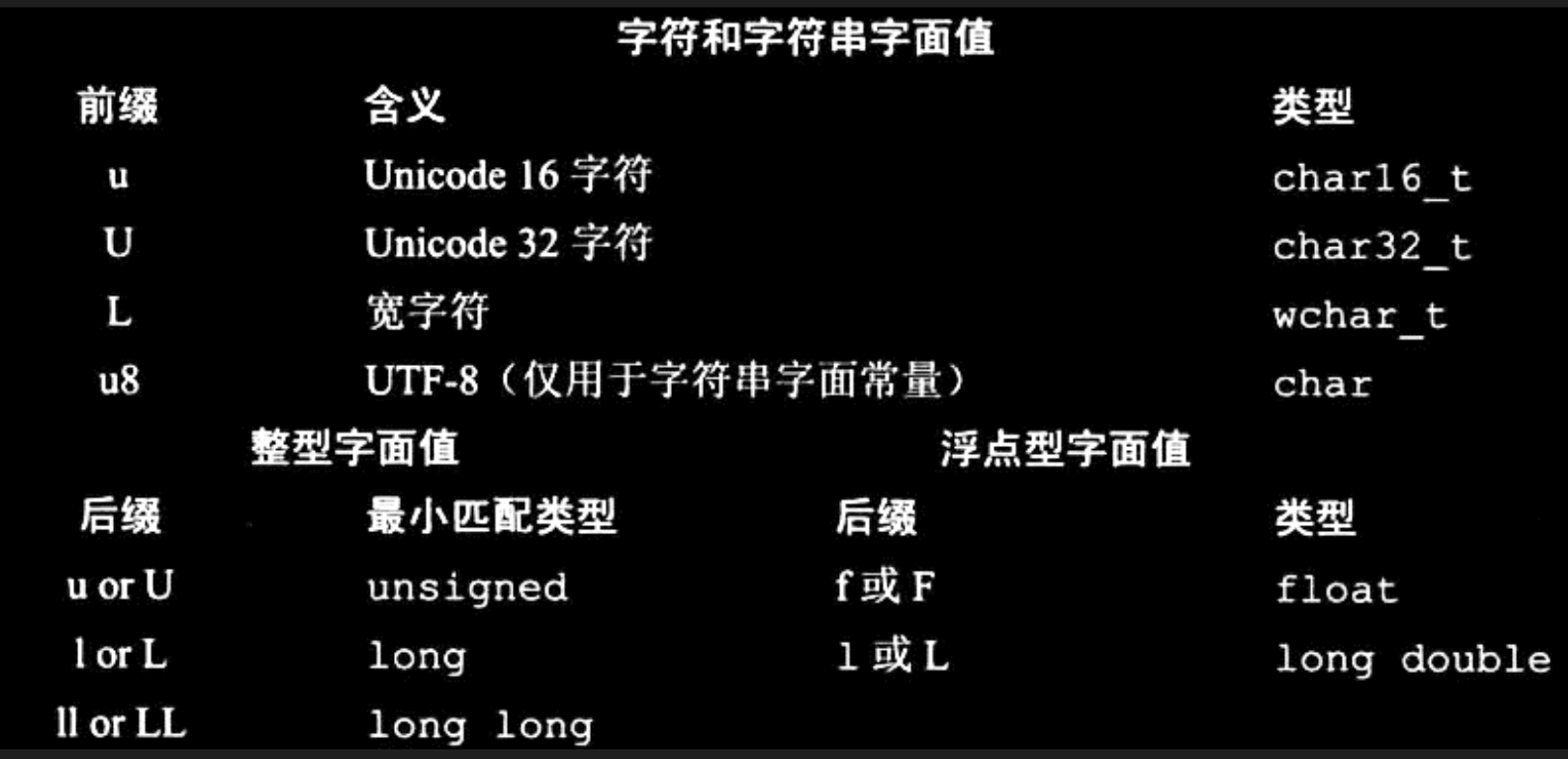``L'a'//宽字符型字面值，类型是wchar_tu8"hi!"  // utf-8 字符串字面值（utf-8 用8 位编码一个 Unicode 字符）42ULL // 无符号整型字面值，类型是 unsigned long long 1E - 3F//单精度浮点型字面值，类型是 float 3.14159L//扩展精度浮点型字面值，类型是 long double//尽量用大写 避免把l看出1``

• 指针 ：`nullptr`
• 布尔：`false true`

# 二、变量

## 概念

• 变量提供一个具有名称的、可供程序操作的存储空间。
• 变量都具有数据类型 • 通过数据类型可以决定变量所需要的内存空间、布局方式、以及能够表示值的范围。

``int sum=0,value,//sum、value和unit_sold都是int    units_sold=0;//sum和units_sold初值为0     Sales_item item;//item的类型是Sales_item    //string 是一种库类型，表示一个可变长的字符序列     std::string book("0-2-1-78345-X");   //book通过一个string字面值初始化``

## 初始化

``int units_sold = 0;//四条语句等价int units_sold = {0};//列表初始化int units_sold{0};//列表初始化 int units_sold(0);long double ld = 3.1415926536; int a{ld},b={ld};//错误：转换未执行，因为存在丢失信息的风险 int c(ld),d=ld;//虽然正确：转换执行，但会丢失精度（部分值）``

• 如果定义变量没有定义初始值，则变量被赋予默认值。
• 默认值是由变量类型决定的，同时定义变量的位置也会有影响。
• 内置类型：由定义的位置决定，函数体之外初始化为`0`
• 每个类各种决定其初始化对象的方式

``std:::string empty;//empty非现实地初始化一个空串Sales_item item;//被默认初始化的Sales_item对象``

## 变量的定义与声明

`C++`是一种静态类型语言，其含义是在编译阶段检查类型。这就要求我们在使用 某个变量之前必须先声明。如果想声明一个变量而非定义它，就在变量名前添加`extern`关键字，而且不要显示的初始化。

``extern double pi = 3.14;//这是定义,不能放在函数体内部,只能放函数外int main() { extern int i;//声明i而非定义i  int j;//声明并定义j}``

## 标识符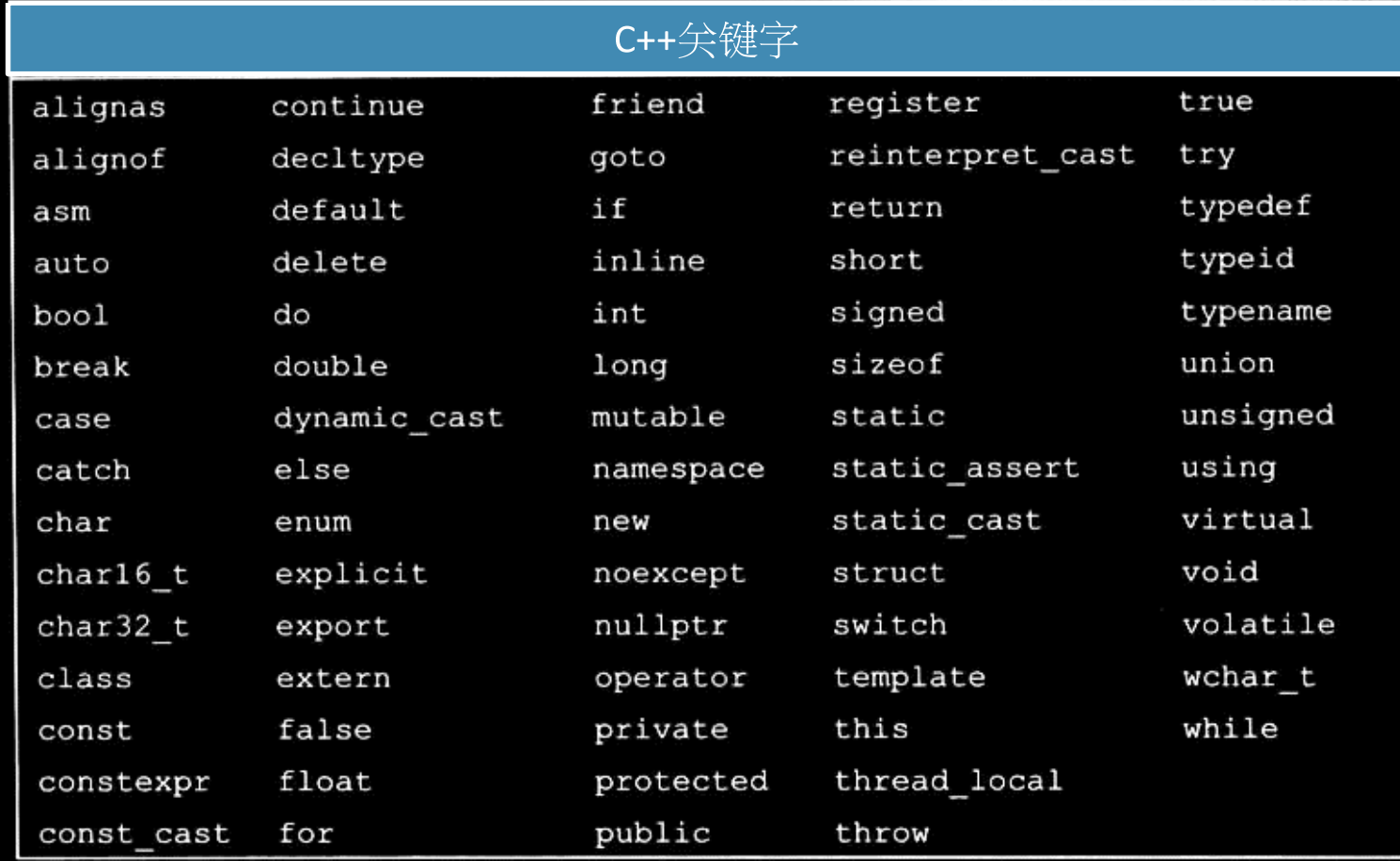## 作用域

``#include  int main(){ //全局作用域int sum = 0;//sum存在全局作用域  它在该区域都能起作用for(int val = 1; val<=10; ++val) {//块作用域 val 只能存在于这个作用域起作用sum+=val;//等价于sum=sum+val}std::cout<<"sum of 1 to 10 inclusive is "<<sum<<std::endl;return 0;}``

``#include // 该程序是一个不好的示例，仅用于展示作用域 int reused = 42; // reused 拥有全局作用域int main() {int unique = 0; // unique 拥有块作用域// output #1: 42 0 std::cout << reused << " " << unique << std::endl; int reused = 0; // 同名的新建局部变量，覆盖了全局变量// output #2: 0 0 std::cout << reused << " " << unique << std::endl;// output #3: 显式地访问全局变量，打印 42 0 std::cout << ::reused << " " << unique << std::endl; return 0;}``

# 三、复合类型

## 引⽤（reference）

C++11中新增了“右值引用”；当我们使用术 语“引用”时，一般指的是“左值引用”。引用不是对象，它只是为一个已经存在的对象所起的另一个名字

``int ival = 1024;int &refVal = ival;//refVal指向ival(是ival的另一个名字)int &refVal2;//报错：引用必须初始化refVal = 2;//把2给refVal指向的对象，此处即是赋给了ival int li = refVal;//等同于li=ival//正确：refVal13绑定到了那个与refVal绑定的对象上，即绑定了ival int &refVal3 = refVal;//利用与refVal绑定的对象的值初始化变量i int i = refVal;//正确：i被初始化为ival的值``

## 指针（pointer）

• 如果一条语句中定义了几个指针变量，每个变量前面都必须加上*符号
• 和其他内置类型一样，在块作用域内定义指针如果没有初始化，将

``int *ip1, *ip2;//ip1和ip2都是指向int型对象的指针 double* dp2;//推荐写法  分行写 并且 类型和*写一起double* dp1;``

•可以使用取地址符（操作符&）获取指针所封装的地址：

``int ival = 42; int *p = &ival;//p是指向ival的指针 double *dp = &ival;//错误：类型不匹配``

•可以使用解引⽤符（操作符*）利用指针访问对象：

``int ival = 42; int *p = &ival;//p是指向ival的指针std::cout<<*p ;//输出42 *p=0;  //等价与 ival = 0 std::cout<<*p; //输出0``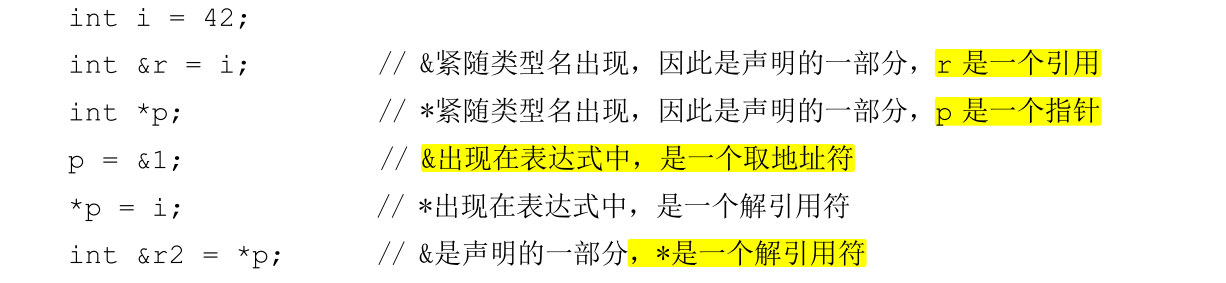## 指针值

• 指针的值（即地址）应属下列 4种状态之一:
1 .指向一个对象。
2 .指向紧邻对象所占空间的下一个位置。
3 .空指针，意味着指针没有指向任何对象。
4 .无效指针，也就是上述情况之外的其他值。
建议：初始化所有指针,如果实在不知道指向哪，就给它赋值为空指针

## 空指针

``int *p1 = nullptr; //C++11  推荐写法int *p2 = 0;int *p3 = NULL; //需要#include cstdlibint zero = 0; p1 = zero; //错误：类型不匹配``

## void *指针

``double obj = 3.14, *pd = &obj; void *pv = &obj;pv = pd;``

## 指向指针的指针

``int ival = 1024; int *pi = &ival; int **ppi = &pi;//ppi指向一个int型的指针``

## 指向指针的引用

``int i = 1024; int* p; int* &r = p; //r是一个对指针p的引用r = &i;//r引用了一个指针，就是令p指向i 等价与 p = &i;*r = 0;//解引用r得到i，也就是p指向的对象，将i的值改为0``

# 四、const 限定符

const限定符：把变量定义成⼀个常量,使用const对变量的类型加以限定，变量的值不能被改变。 防止出现风险

## 初始化

const对象必须初始化（其他时候不能出现在等号左边）。

``const int bufSize = 512; //输入缓冲区大小bufSize = 512; //错误：试图向const对象写值const int i = get_size(); //正确：运行时初始化 const int j = 42; //正确：编译时初始化 const int k; //错误：k是一个未经初始化的常量const int bb=0; void * a= bb;//在编译的时候，会把bb编程常量``

``//file_1.cc定义并初始化了一个常量，该常量能被其他文件访问 extern const int bufSize = fcn(); //file_1.h头文件 中声明extern const int bufSize;``

## const的引⽤：常量引用

``const int ci = 1024; const int &r1 = ci; //正确：引用及其绑定的对象都是常量 （常量引用）r1 = 42;//错误，相当于c1=42，试图修改常量 int &r2 = ci;//错误：ci是常量，存在通过r2改变ci（const）的风险int i = 42; const int &r1 = i; //正确：i依然可以通过其他途径修改 const int &r2 = 42; const int &r3 = r1*2; int &r4 = r1*2; //错误：不能通过一个非常量的引用指向一个常量``

``double dval = 3.14;const int &ri = dval; //这个语句实际上会运行为 以下俩条语句组成const int tmep = dval;//把double 转化为 int 类型常量const int &ri = temp;``

## 指针和const

``const double pi = 3.14;double *ptr = &pi; //错误：存在通过ptr指针修改pi的风险const double * cptr = &pi;  //相当于限制了解引用操作*cptr = 42; //错误double dval = 3.14; cptr = &dval; //正确：但不能通过cptr修改dval的值  改地址不影响``

const指针：指针是对象，也可以限定为常量（必须初始化）
• 把`*`放在`const`之前，说明指针是一个常量
• 不变的是指针本身的值，而非指向的那个值

``int errNumb = 0; int *const curErr = &errNumb; const double pi = 3.14159; const double *const pip = &pi;//指向常量的常量指针*pip = 2.71;//错误： 试图修改常量piif(*curErr){ errorHandler();*curErr = 0; //正确：试图修改变量errNumb}``

## 顶层const & 底层const

• 顶层const：表示变量本身是一个常量
• 底层const：表示指针所指向的对象是一个const

``int i = 0; int *const p1 = &i; //顶层 const int ci = 42; //顶层 const int *p2 = &ci;//底层 const int *const p3 = p2;//（左：底层 ）， （右：顶层）i = ci;//正确 p2 = p3;//正确int *p = p3;//错误：存在通过*p修改*p3（const）的风险 p2 = &i; //正确：只是不能通过p2修改i而已 int &r = ci; //错误：存在通过r修改ci（const)的风险 const int &r2 = i; //正确：只是不能通过r2修改i而已``

## constexpr和常量表达式

• 常量表达式（const expression）是指：值不会改变并且在编译过程就能得到计算结果的表达式。自定义类型（例如：Sales_item）、IO库、 string等类型不能被定义为constexpr。
• C++11标准规定，允许将变量声明为constexpr类型，以便由编译器来验证变量的值是否是一个常量表达式。
• 一定是一个常量
• 必须用常量表达式初始化

``constexpr int mf = 20; constexpr int limit = mf +1; constexpr int sz = size(); //只有当size是一个constexpr函数时才正确``

• 限定符仅对指针有效，对指针所指的对象无关

``// i j 必须定义在函数体外int j = 0; constexpr int i = 42;int main() {    constexpr int *np = nullptr; //常量指针     constexpr const int *p = &i; //p是常量指针，指向常量     constexpr int *p1 = &j; //p1是常量指针，指向变量j    return 0;}   ``

# 五、处理类型

• 拼写变得越来越困难。 -> typedef
• 搞不清楚变量到底需要什么类型。 ->auto

## typedef

``typedef double wages; typedef wages base, *p; //base是double的同义词，p是double *的同义词using SI = Sales_item; //C++11,别名声明wages hourly, weekly; //等价于double hourly, weeklySI item; //等价于Sales_item item``

``typedef char *pstring;   //pstring 是 char* 的同义词const pstring cstr = 0; //指向char的常量指针 const pstring *ps; //ps是指针变量，它的对象是指向char的常量指针const char *cstr = 0; //是对const pstring cstr =0 的错误理解``

## auto

atuo类型说明符：C++11，让编译器通过初始值推断变量的类型

``auto item = val1 + val2;auto i =0, *p = &i; //正确 auto sz = 0, pi = 3.14; //错误：auto已经被推断为int型，却需要被推断为double``

auto会忽略顶层const

``int i = 0, &r = i; auto a = r; //a是int型const int ci = i, &cr = ci; auto b = ci; //b是int型，ci的顶层const被忽略 auto c = cr; //c是int型，ci的顶层const被忽略 auto d = &i; //d是整形指针，整数的地址就是指向整形的指针 auto e = &ci; //e是指向整数常量的指针（底层const没有被忽略）const auto f = ci; //auto的推演类型为 int，f是const int auto &g = ci; //g是一个整形常量引用，绑定到ci，（底层const没有被忽略）auto &h = 42; //错误：不能为非常量引用绑定字面值 const auto &j =42; //正确：可以为常量引用绑定字面值auto k = ci, &l = i;  //k 是整数 ， l是整型引用auto &m = ci, *p = &ci; //m 是对整型常量的引用  p是指向整型常量的指针auto &n = i, *p2 = &ci; //errot : i是int   &ci 是const int 类型不同``

## decltype

decltype类型说明符：选择并返回操作数的数据类型 只要数据类型，不要其值

``decltype(f()) sum = x;// sum的类型就是函数f返回的类型const int ci = 0, &cj = ci; decltype(ci) x = 0; // x的类型是const int decltype(cj) y = x; // y的类型是const int & decltype(cj) z; //错误：z是一个引用，必须初始化``

*decltype和引用

decltype((variable))：双层括号，结果永远是引用。 多一层()相当于 加上&

``int i = 42, *p = &i, &r = i; decltype(r+0) b; //正确：b为int型//注意：下面的*不是出现在声明中，而是表达式中的解引用运算符 decltype(*p) c; //错误：解引用表达式，c的类型为引用int &，需要初始化//变量如果是加上括号， decltype的结果将是引用 decltype( (i) ) d; //错误：d是int&类型,必须初始化 decltype( ( (i) ) ) d1 = i; //正确：d1是int&类型，绑定为了i decltype( i ) e; //正确：e是一个（未初始化的）int``

# 六、自定义数据结构

## 类型

⾃定义数据结构：⼀组数据以及相关操作的集合

• 二者默认的继承访问权限不同
`struct``public`的，`class``private`
•结尾分号不可少！！

``struct Sales_data{ std::string bookNo; unsigned units_sold = 0; //C++ 11 double revenue = 0.0;};//类定义的最后需要加上分号``

`Sales_data accum,trans,*salesptr;`

## 类的使用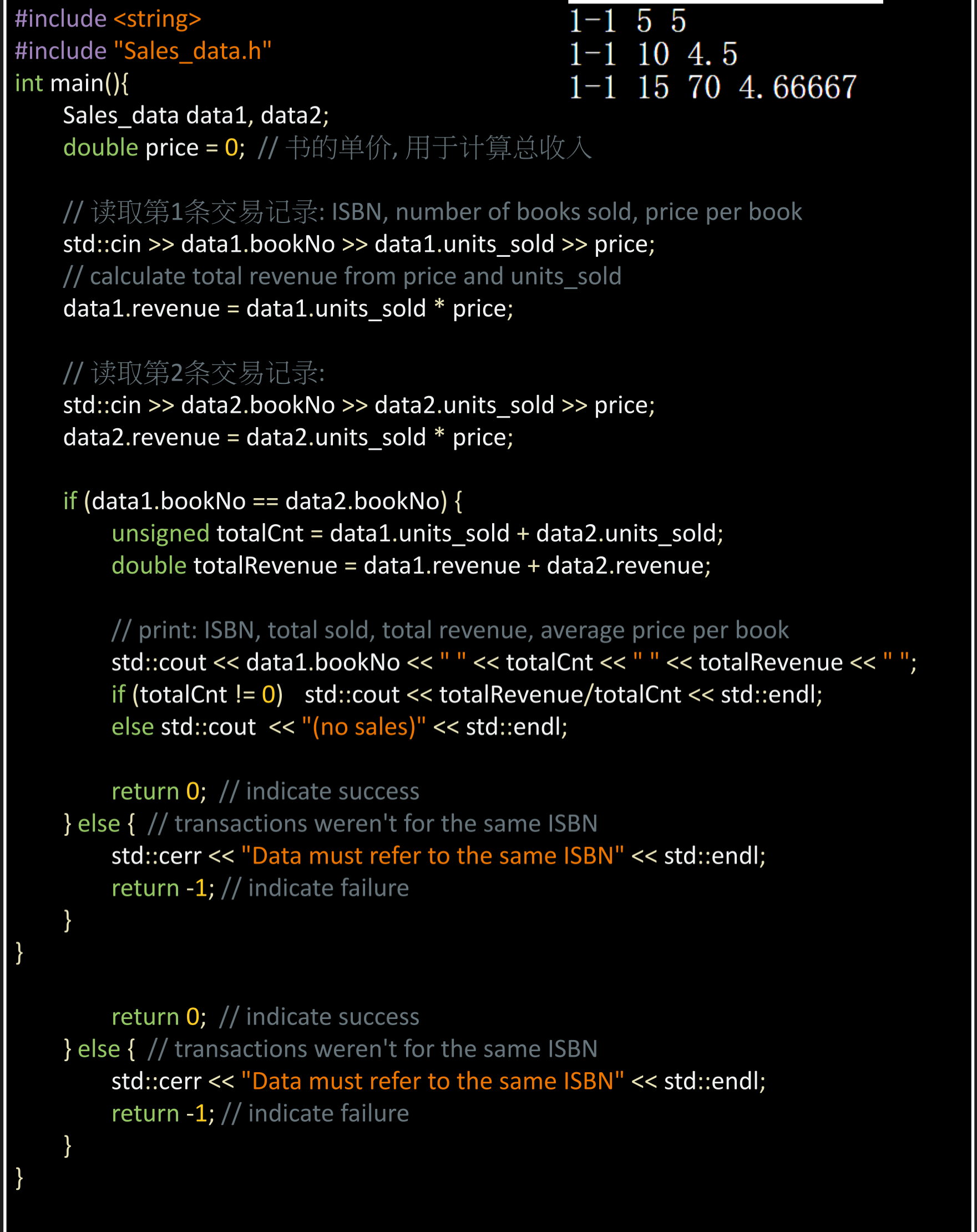## 头文件 “xx.h”

``//预处理器（preprocessor）， 在编译之前执行的代码。// 这里预处理器的功能是头文件保护符。//其作用是防止重复包含的发生，只会调用一次"string.h"#ifndef SALES_DATA_H #define SALES_DATA_H#include struct Sales_data { std::string bookNo; unsigned units_sold = 0; double revenue = 0.0;}; #endif``

`#include `实际也是预处理,当预处理器看到`#include` 标记时就会用指定的头文件的内容代替`#include`

# ?感谢阅读?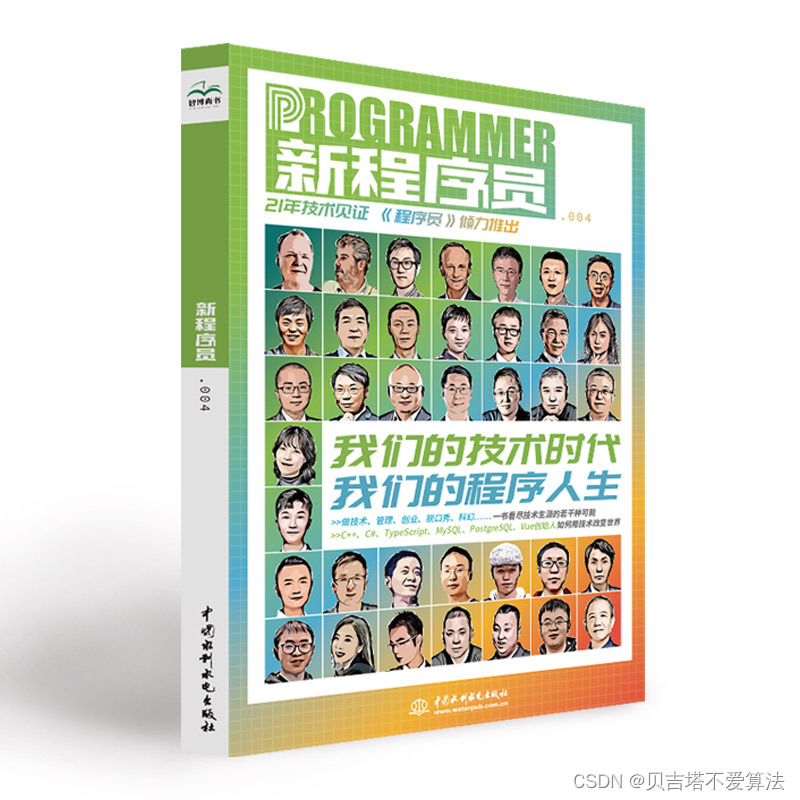开发者涨薪指南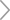48位大咖的思考法则、工作方式、逻辑体系海量搞笑GIF动态图片